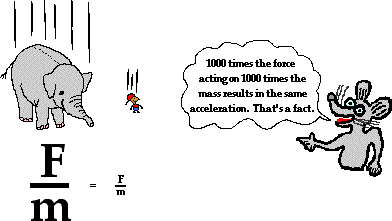Free Fall and the Acceleration of Gravity
04-19-2010

Introduction to Free Fall

A free-falling object is an object which is falling under the sole influence of gravity. Any object which is being acted upon only be the force of gravity is said to be in a state of free fall. There are two important motion characteristics which are true of free-falling objects:
• Free-falling objects do not encounter air resistance.
• All free-falling objects (on Earth) accelerate downwards at a rate of 9.8 m/s/s (often approximated as 10 m/s/s for back-of-the-envelope calculations)Because free-falling objects are accelerating downwards at a rate of 9.8 m/s/s, a ticker tape trace or dot diagram of its motion would depict an acceleration. The dot diagram at the right depicts the acceleration of a free-falling object. The position of the object at regular time intervals - say, every 0.1 second - is shown. The fact that the distance which the object travels every interval of time is increasing is a sure sign that the ball is speeding up as it falls downward. Recall from an earlier lesson, that if an object travels downward and speeds up, then its acceleration is downward.
Free-fall acceleration is often witnessed in a physics classroom by means of an ever-popular strobe light demonstration. The room is darkened and a jug full of water is connected by a tube to a medicine dropper. The dropper drips water and the strobe illuminates the falling droplets at a regular rate - say once every 0.2 seconds. Instead of seeing a stream of water free-falling from the medicine dropper, several consecutive drops with increasing separation distance are seen. The pattern of drops resembles the dot diagram shown in the graphic at the right

The Acceleration of Gravity

It was learned in the previous part of this lesson that a free-falling object is an object which is falling under the sole influence of gravity. A free-falling object has an acceleration of 9.8 m/s/s, downward (on Earth). This numerical value for the acceleration of a free-falling object is such an important value that it is given a special name. It is known as theacceleration of gravity - the acceleration for any object moving under the sole influence of gravity. A matter of fact, this quantity known as the acceleration of gravity is such an important quantity that physicists have a special symbol to denote it - the symbol g. The numerical value for the acceleration of gravity is most accurately known as 9.8 m/s/s. There are slight variations in this numerical value (to the second decimal place) which are dependent primarily upon on altitude. We will occasionally use the approximated value of 10 m/s/s in The Physics Classroom Tutorial in order to reduce the complexity of the many mathematical tasks which we will perform with this ******. By so doing, we will be able to better focus on the conceptual nature of physics without too much of a sacrifice in numerical accuracy.

g = 9.8 m/s/s, downward
( ~ 10 m/s/s, downward)
Recall from an earlier lesson that acceleration is the rate at which an object changes its velocity. It is the ratio of velocity change to time between any two points in an object's path. To accelerate at 9.8 m/s/s means to change the velocity by 9.8 m/s each second.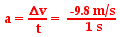If the velocity and time for a free-falling object being dropped from a position of rest were tabulated, then one would note the following pattern.
Time (s)Velocity (m/s)001- 9.82- 19.63- 29.44- 39.25- 49.0Observe that the velocity-time data above reveal that the object's velocity is changing by 9.8 m/s each consecutive second. That is, the free-falling object has an acceleration of approximately 9.8 m/s/s.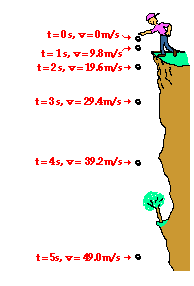Another way to represent this acceleration of 9.8 m/s/s is to add ******s to our dot diagram which we saw earlier in this lesson. The velocity of the ball is seen to increase as depicted in the diagram at the right. (NOTE: The diagram is not drawn to scale - in two seconds, the object would drop considerably further than the distance from shoulder to toes

Representing Free Fall by Graphs

Early in Lesson 1 it was mentioned that there are a variety of means of describing the motion of objects. One such means of describing the motion of objects is through the use of graphs - position versus time and velocity vs. time graphs. In this part of Lesson 5, the motion of a free-falling motion will be represented using these two basic types of graphs.
A position versus time graph for a free-falling object is shown below.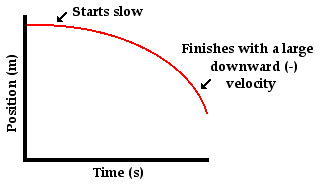Observe that the line on the graph curves. As learned earlier, a curved line on a position versus time graph signifies an accelerated motion. Since a free-falling object is undergoing an acceleration (g = 9.8 m/s/s), it would be expected that its position-time graph would be curved. A further look at the position-time graph reveals that the object starts with a small velocity (slow) and finishes with a large velocity (fast). Since the slope of any position vs. time graph is the velocity of the object (as learned in Lesson 3), the small initial slope indicates a small initial velocity and the large final slope indicates a large final velocity. Finally, the negative slope of the line indicates a negative (i.e., downward) velocity.
A velocity versus time graph for a free-falling object is shown below.Observe that the line on the graph is a straight, diagonal line. As learned earlier, a diagonal line on a velocity versus time graph signifies an accelerated motion. Since a free-falling object is undergoing an acceleration (g = 9,8 m/s/s, downward), it would be expected that its velocity-time graph would be diagonal. A further look at the velocity-time graph reveals that the object starts with a zero velocity (as read from the graph) and finishes with a large, negative velocity; that is, the object is moving in the negative direction and speeding up. An object which is moving in the negative direction and speeding up is said to have a negative acceleration (if necessary, review the vector nature of acceleration). Since the slope of any velocity versus time graph is the acceleration of the object (as learned in Lesson 4), the constant, negative slope indicates a constant, negative acceleration. This analysis of the slope on the graph is consistent with the motion of a free-falling object - an object moving with a constant acceleration of 9.8 m/s/s in the downward direction.
How Fast? and How Far?Free-falling objects are in a state of acceleration. Specifically, they are accelerating at a rate of 9.8 m/s/s. This is to say that the velocity of a free-falling object is changing by 9.8 m/s every second. If dropped from a position of rest, the object will be traveling 9.8 m/s (approximately 10 m/s) at the end of the first second, 19.6 m/s (approximately 20 m/s) at the end of the second second, 29.4 m/s (approximately 30 m/s) at the end of the third second, etc. Thus, the velocity of a free-falling object which has been dropped from a position of rest is dependent upon the time for which it has fallen. The formula for determining the velocity of a falling object after a time of t seconds is
vf = g * t

where g is the acceleration of gravity. The value for g on Earth is 9.8 m/s/s. The above equation can be used to calculate the velocity of the object after any given amount of time when dropped from rest. Example calculations for the velocity of a free-falling object after six and eight seconds are shown below.
Example Calculations:

At t = 6 s
vf = (9.8 m/s2) * (6 s) = 58.8 m/s
At t = 8 s
vf = (9.8 m/s2) * (8 s) = 78.4 m/s
The distance which a free-falling object has fallen from a position of rest is also dependent upon the time of fall. This distance can be computed by use of a formula; the distance fallen after a time of t seconds is given by the formula.
d = 0.5 * g * t2

where g is the acceleration of gravity (9.8 m/s/s on Earth). Example calculations for the distance fallen by a free-falling object after one and two seconds are shown below.
Example Calculations:

At t = 1 s
d = (0.5) * (9.8 m/s2) * (1 s)2 = 4.9 m
At t = 2 s
d = (0.5) * (9.8 m/s2) * (2 s)2 = 19.6 m
At t = 5 s
d = (0.5) * (10 m/s2) * (5 s)2 = 123 m
(rounded from 122.5 m)
The diagram below (not drawn to scale) shows the results of several distance calculations for a free-falling object dropped from a position of rest.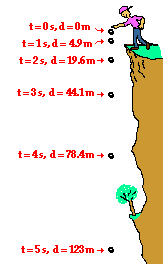The Big MisconceptionEarlier in this lesson, it was stated that the acceleration of a free-falling object (on earth) is 9.8 m/s/s. This value (known as the acceleration of gravity) is the same for all free-falling objects regardless of how long they have been falling, or whether they were initially dropped from rest or thrown up into the air. Yet the questions are often asked "doesn't a more massive object accelerate at a greater rate than a less massive object?" "Wouldn't an elephant free-fall faster than a mouse?" This question is a reasonable inquiry that is probably based in part upon personal observations made of falling objects in the physical world. After all, nearly everyone has observed the difference in the rate of fall of a single piece of paper (or similar object) and a textbook. The two objects clearly travel to the ground at different rates - with the more massive book falling faster.
The answer to the question (doesn't a more massive object accelerate at a greater rate than a less massive object?) is absolutely not! That is, absolutely not if we are considering the specific type of falling motion known as free-fall. Free-fall is the motion of objects which move under the sole influence of gravity; free-falling objects do not encounter air resistance. More massive objects will only fall faster if there is an appreciable amount of air resistance present.
The actual explanation of why all objects accelerate at the same rate involves the concepts of force and mass. The details will be discussed in Unit 2 of The Physics Classroom. At that time, you will learn that the acceleration of an object is directly proportional to force and inversely proportional to mass. Increasing force tends to increase acceleration while increasing mass tends to decrease acceleration. Thus, the greater force on more massive objects is offset by the inverse influence of greater mass. Subsequently, all objects free fall at the same rate of acceleration, regardless of their mass.